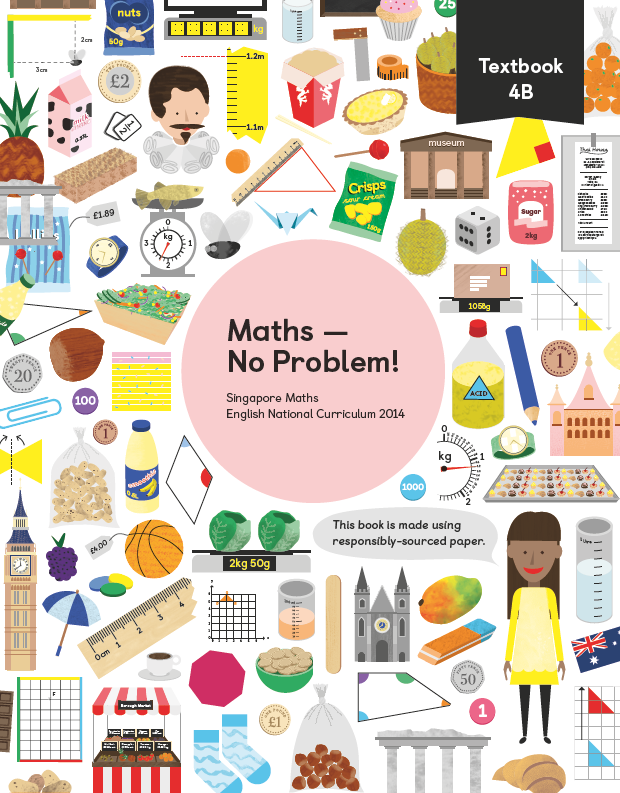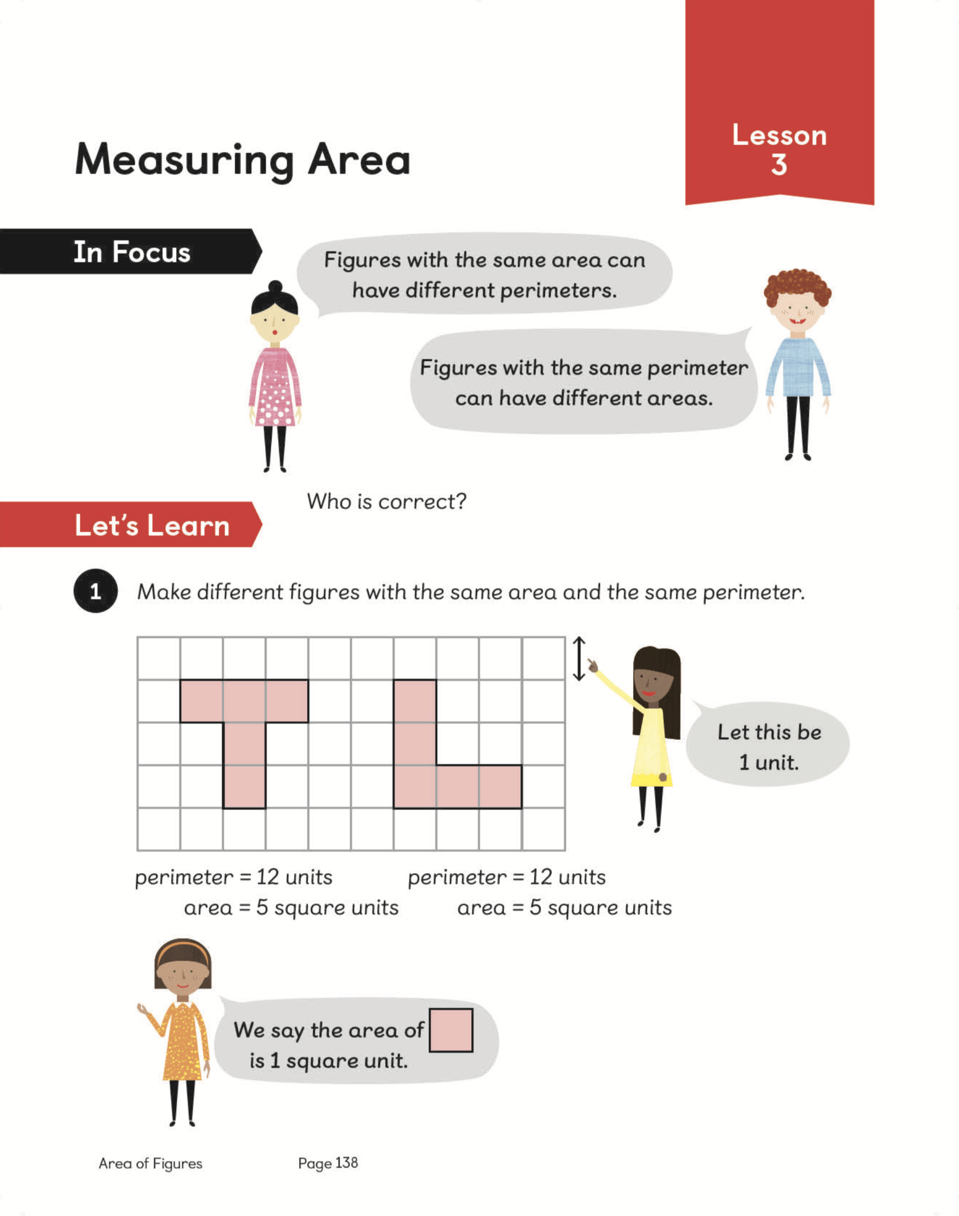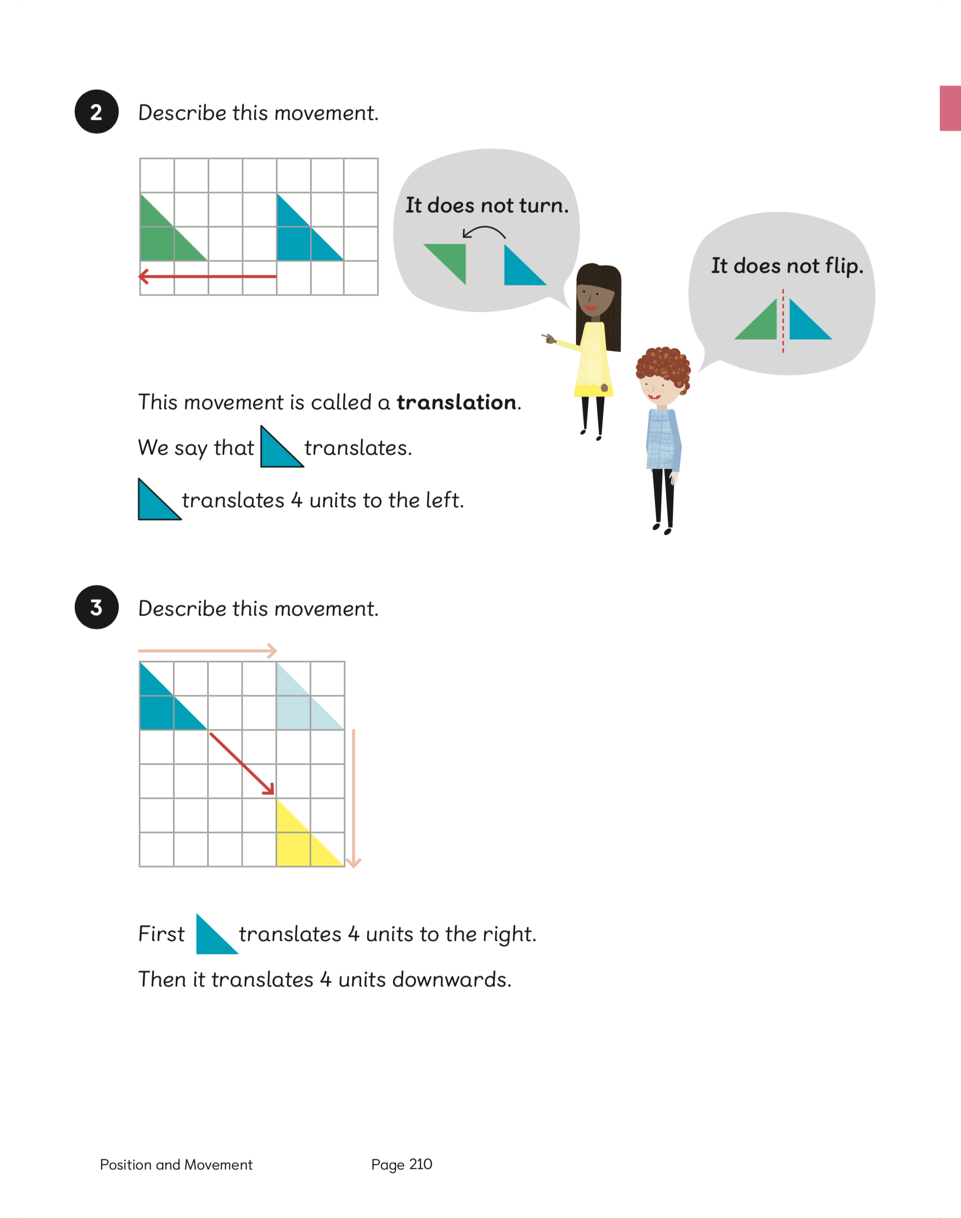Textbook 4B# Maths — No Problem!Textbook 4B

## £9.99

ISBN: 9781910504185
Pages: 208
Availability:
In stock
Qty:

• Lesson 1 - Writing Tenths

To be able to recognise and write decimal equivalents of any number of tenths.

• Lesson 2 - Writing Tenths

To be able to recognise and write decimal equivalents of any number of tenths.

• Lesson 3 - Writing Tenths

To be able to recognise and write decimal equivalents of any number of tenths.

• Lesson 4 - Writing Hundredths

To be able to recognise and write decimal equivalents of any number of hundredths.

• Lesson 5 - Writing Hundredths

To be able to recognise and write decimal equivalents of any number of hundredths.

• Lesson 6 - Writing Hundredths

To be able to recognise and write decimal equivalents of any number of hundredths.

• Lesson 7 - Writing Hundredths

To be able to recognise and write decimal equivalents of any number of hundredths.

• Lesson 8 - Writing Decimals

To be able to read and write numbers as decimals.

• Lesson 9 - Comparing and Ordering Decimals

To be able to compare and order numbers with the same number of decimal places up to 2 decimal places.

• Lesson 10 - Comparing and Ordering Decimals

To be able to compare and order numbers with the same number of decimal places up to 2 decimal places.

• Lesson 11 - Comparing and Ordering Decimals

To be able to compare and order numbers with the same number of decimal places up to 2 decimal places.

• Lesson 12 - Making Number Patterns

To be able to identify numbers, which are 1 tenth or 1 hundredth more/less in a number sequence.

• Lesson 13 - Rounding Decimals

To be able to round numbers with 1 decimal place to the nearest whole number.

• Lesson 14 - Rounding Decimals

To be able to round numbers with 1 decimal place to the nearest whole number.

• Lesson 15 - Writing Fractions as Decimals

To be able to recognise and write decimal equivalents to 1⁄4, 1⁄2, 3⁄4.

• Lesson 16 - Dividing Whole Numbers by 10

To be able to divide 1- or 2-digit numbers by 10.

• Lesson 17 - Dividing Whole Numbers by 100

To be able to divide 1- or 2-digit numbers by 100.

• Lesson 18 - Chapter Consolidation

To be able to apply knowledge of decimal numbers to solve problems.

## Author(s)

Consultant and Author: Dr. Yeap Ban Har
UK Consultant: Dr. Anne Hermanson